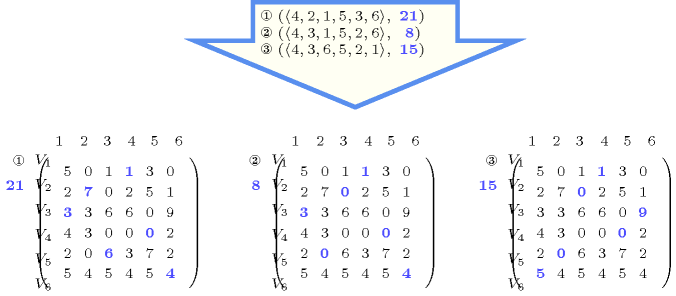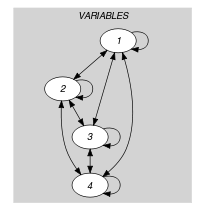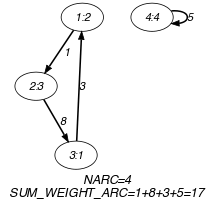## 5.266. minimum_weight_alldifferent

Origin
Constraint

$\mathrm{𝚖𝚒𝚗𝚒𝚖𝚞𝚖}_\mathrm{𝚠𝚎𝚒𝚐𝚑𝚝}_\mathrm{𝚊𝚕𝚕𝚍𝚒𝚏𝚏𝚎𝚛𝚎𝚗𝚝}\left(\mathrm{𝚅𝙰𝚁𝙸𝙰𝙱𝙻𝙴𝚂},\mathrm{𝙼𝙰𝚃𝚁𝙸𝚇},\mathrm{𝙲𝙾𝚂𝚃}\right)$

Synonyms

$\mathrm{𝚖𝚒𝚗𝚒𝚖𝚞𝚖}_\mathrm{𝚠𝚎𝚒𝚐𝚑𝚝}_\mathrm{𝚊𝚕𝚕𝚍𝚒𝚏𝚏}$, $\mathrm{𝚖𝚒𝚗𝚒𝚖𝚞𝚖}_\mathrm{𝚠𝚎𝚒𝚐𝚑𝚝}_\mathrm{𝚊𝚕𝚕𝚍𝚒𝚜𝚝𝚒𝚗𝚌𝚝}$, $\mathrm{𝚖𝚒𝚗}_\mathrm{𝚠𝚎𝚒𝚐𝚑𝚝}_\mathrm{𝚊𝚕𝚕𝚍𝚒𝚏𝚏}$, $\mathrm{𝚖𝚒𝚗}_\mathrm{𝚠𝚎𝚒𝚐𝚑𝚝}_\mathrm{𝚊𝚕𝚕𝚍𝚒𝚏𝚏𝚎𝚛𝚎𝚗𝚝}$, $\mathrm{𝚖𝚒𝚗}_\mathrm{𝚠𝚎𝚒𝚐𝚑𝚝}_\mathrm{𝚊𝚕𝚕𝚍𝚒𝚜𝚝𝚒𝚗𝚌𝚝}$.

Arguments
 $\mathrm{𝚅𝙰𝚁𝙸𝙰𝙱𝙻𝙴𝚂}$ $\mathrm{𝚌𝚘𝚕𝚕𝚎𝚌𝚝𝚒𝚘𝚗}\left(\mathrm{𝚟𝚊𝚛}-\mathrm{𝚍𝚟𝚊𝚛}\right)$ $\mathrm{𝙼𝙰𝚃𝚁𝙸𝚇}$ $\mathrm{𝚌𝚘𝚕𝚕𝚎𝚌𝚝𝚒𝚘𝚗}\left(𝚒-\mathrm{𝚒𝚗𝚝},𝚓-\mathrm{𝚒𝚗𝚝},𝚌-\mathrm{𝚒𝚗𝚝}\right)$ $\mathrm{𝙲𝙾𝚂𝚃}$ $\mathrm{𝚍𝚟𝚊𝚛}$
Restrictions
 $|\mathrm{𝚅𝙰𝚁𝙸𝙰𝙱𝙻𝙴𝚂}|>0$ $\mathrm{𝚛𝚎𝚚𝚞𝚒𝚛𝚎𝚍}$$\left(\mathrm{𝚅𝙰𝚁𝙸𝙰𝙱𝙻𝙴𝚂},\mathrm{𝚟𝚊𝚛}\right)$ $\mathrm{𝚅𝙰𝚁𝙸𝙰𝙱𝙻𝙴𝚂}.\mathrm{𝚟𝚊𝚛}\ge 1$ $\mathrm{𝚅𝙰𝚁𝙸𝙰𝙱𝙻𝙴𝚂}.\mathrm{𝚟𝚊𝚛}\le |\mathrm{𝚅𝙰𝚁𝙸𝙰𝙱𝙻𝙴𝚂}|$ $\mathrm{𝚛𝚎𝚚𝚞𝚒𝚛𝚎𝚍}$$\left(\mathrm{𝙼𝙰𝚃𝚁𝙸𝚇},\left[𝚒,𝚓,𝚌\right]\right)$ $\mathrm{𝚒𝚗𝚌𝚛𝚎𝚊𝚜𝚒𝚗𝚐}_\mathrm{𝚜𝚎𝚚}$$\left(\mathrm{𝙼𝙰𝚃𝚁𝙸𝚇},\left[𝚒,𝚓\right]\right)$ $\mathrm{𝙼𝙰𝚃𝚁𝙸𝚇}.𝚒\ge 1$ $\mathrm{𝙼𝙰𝚃𝚁𝙸𝚇}.𝚒\le |\mathrm{𝚅𝙰𝚁𝙸𝙰𝙱𝙻𝙴𝚂}|$ $\mathrm{𝙼𝙰𝚃𝚁𝙸𝚇}.𝚓\ge 1$ $\mathrm{𝙼𝙰𝚃𝚁𝙸𝚇}.𝚓\le |\mathrm{𝚅𝙰𝚁𝙸𝙰𝙱𝙻𝙴𝚂}|$ $|\mathrm{𝙼𝙰𝚃𝚁𝙸𝚇}|=|\mathrm{𝚅𝙰𝚁𝙸𝙰𝙱𝙻𝙴𝚂}|*|\mathrm{𝚅𝙰𝚁𝙸𝙰𝙱𝙻𝙴𝚂}|$
Purpose

All variables of the $\mathrm{𝚅𝙰𝚁𝙸𝙰𝙱𝙻𝙴𝚂}$ collection should take a distinct value located within interval $\left[1,|\mathrm{𝚅𝙰𝚁𝙸𝙰𝙱𝙻𝙴𝚂}|\right]$. In addition $\mathrm{𝙲𝙾𝚂𝚃}$ is equal to the sum of the costs associated with the fact that we assign value $i$ to variable $j$. These costs are given by the matrix $\mathrm{𝙼𝙰𝚃𝚁𝙸𝚇}$.

Example
$\left(\begin{array}{c}〈2,3,1,4〉,\hfill \\ 〈\begin{array}{ccc}𝚒-1\hfill & 𝚓-1\hfill & 𝚌-4,\hfill \\ 𝚒-1\hfill & 𝚓-2\hfill & 𝚌-1,\hfill \\ 𝚒-1\hfill & 𝚓-3\hfill & 𝚌-7,\hfill \\ 𝚒-1\hfill & 𝚓-4\hfill & 𝚌-0,\hfill \\ 𝚒-2\hfill & 𝚓-1\hfill & 𝚌-1,\hfill \\ 𝚒-2\hfill & 𝚓-2\hfill & 𝚌-0,\hfill \\ 𝚒-2\hfill & 𝚓-3\hfill & 𝚌-8,\hfill \\ 𝚒-2\hfill & 𝚓-4\hfill & 𝚌-2,\hfill \\ 𝚒-3\hfill & 𝚓-1\hfill & 𝚌-3,\hfill \\ 𝚒-3\hfill & 𝚓-2\hfill & 𝚌-2,\hfill \\ 𝚒-3\hfill & 𝚓-3\hfill & 𝚌-1,\hfill \\ 𝚒-3\hfill & 𝚓-4\hfill & 𝚌-6,\hfill \\ 𝚒-4\hfill & 𝚓-1\hfill & 𝚌-0,\hfill \\ 𝚒-4\hfill & 𝚓-2\hfill & 𝚌-0,\hfill \\ 𝚒-4\hfill & 𝚓-3\hfill & 𝚌-6,\hfill \\ 𝚒-4\hfill & 𝚓-4\hfill & 𝚌-5\hfill \end{array}〉,17\hfill \end{array}\right)$

The $\mathrm{𝚖𝚒𝚗𝚒𝚖𝚞𝚖}_\mathrm{𝚠𝚎𝚒𝚐𝚑𝚝}_\mathrm{𝚊𝚕𝚕𝚍𝚒𝚏𝚏𝚎𝚛𝚎𝚗𝚝}$ constraint holds since the cost 17 corresponds to the sum $\mathrm{𝙼𝙰𝚃𝚁𝙸𝚇}\left[\left(1-1\right)·4+2\right].𝚌+\mathrm{𝙼𝙰𝚃𝚁𝙸𝚇}\left[\left(2-1\right)·4+3\right].𝚌+\mathrm{𝙼𝙰𝚃𝚁𝙸𝚇}\left[\left(3-1\right)·4+1\right].𝚌+\mathrm{𝙼𝙰𝚃𝚁𝙸𝚇}\left[\left(4-1\right)·4+4\right].𝚌=\mathrm{𝙼𝙰𝚃𝚁𝙸𝚇}\left[2\right].𝚌+\mathrm{𝙼𝙰𝚃𝚁𝙸𝚇}\left[7\right].𝚌+\mathrm{𝙼𝙰𝚃𝚁𝙸𝚇}\left[9\right].𝚌+\mathrm{𝙼𝙰𝚃𝚁𝙸𝚇}\left[16\right].𝚌=1+8+3+5$.

All solutions

Figure 5.266.1 gives all solutions to the following non ground instance of the $\mathrm{𝚖𝚒𝚗𝚒𝚖𝚞𝚖}_\mathrm{𝚠𝚎𝚒𝚐𝚑𝚝}_\mathrm{𝚊𝚕𝚕𝚍𝚒𝚏𝚏𝚎𝚛𝚎𝚗𝚝}$ constraint:

${V}_{1}\in \left[2,4\right]$, ${V}_{2}\in \left[2,3\right]$, ${V}_{3}\in \left[1,6\right]$, ${V}_{4}\in \left[2,5\right]$, ${V}_{5}\in \left[2,3\right]$, ${V}_{6}\in \left[1,6\right]$, $C\in \left[0,25\right]$,

$\mathrm{𝚖𝚒𝚗𝚒𝚖𝚞𝚖}_\mathrm{𝚠𝚎𝚒𝚐𝚑𝚝}_\mathrm{𝚊𝚕𝚕𝚍𝚒𝚏𝚏𝚎𝚛𝚎𝚗𝚝}$$\left(〈{V}_{1},{V}_{2},{V}_{3},{V}_{4},{V}_{5},{V}_{6}〉$,

$〈115,120,131,141,153,160$

$212,227,230,242,255,261$

$313,323,336,346,350,369$

$414,423,430,440,450,462$

$512,520,536,543,557,562$

$615,624,635,644,655,664〉,C\right)$.

##### Figure 5.266.1. All solutions corresponding to the non ground example of the $\mathrm{𝚖𝚒𝚗𝚒𝚖𝚞𝚖}_\mathrm{𝚠𝚎𝚒𝚐𝚑𝚝}_\mathrm{𝚊𝚕𝚕𝚍𝚒𝚏𝚏𝚎𝚛𝚎𝚗𝚝}$ constraint of the All solutions slotTypical
 $|\mathrm{𝚅𝙰𝚁𝙸𝙰𝙱𝙻𝙴𝚂}|>1$ $\mathrm{𝚛𝚊𝚗𝚐𝚎}$$\left(\mathrm{𝙼𝙰𝚃𝚁𝙸𝚇}.𝚌\right)>1$ $\mathrm{𝙼𝙰𝚃𝚁𝙸𝚇}.𝚌>0$
Arg. properties

Functional dependency: $\mathrm{𝙲𝙾𝚂𝚃}$ determined by $\mathrm{𝚅𝙰𝚁𝙸𝙰𝙱𝙻𝙴𝚂}$ and $\mathrm{𝙼𝙰𝚃𝚁𝙸𝚇}$.

Algorithm

The Hungarian method for the assignment problem [Kuhn55] can be used for evaluating the bounds of the $\mathrm{𝙲𝙾𝚂𝚃}$ variable. A filtering algorithm is described in [Sellmann02]. It can be used for handling both side of the $\mathrm{𝚖𝚒𝚗𝚒𝚖𝚞𝚖}_\mathrm{𝚠𝚎𝚒𝚐𝚑𝚝}_\mathrm{𝚊𝚕𝚕𝚍𝚒𝚏𝚏𝚎𝚛𝚎𝚗𝚝}$ constraint:

• Evaluating a lower bound of the $\mathrm{𝙲𝙾𝚂𝚃}$ variable and pruning the variables of the $\mathrm{𝚅𝙰𝚁𝙸𝙰𝙱𝙻𝙴𝚂}$ collection in order to not exceed the maximum value of $\mathrm{𝙲𝙾𝚂𝚃}$.

• Evaluating an upper bound of the $\mathrm{𝙲𝙾𝚂𝚃}$ variable and pruning the variables of the $\mathrm{𝚅𝙰𝚁𝙸𝙰𝙱𝙻𝙴𝚂}$ collection in order to not be under the minimum value of $\mathrm{𝙲𝙾𝚂𝚃}$.

Systems
Keywords
Arc input(s)

$\mathrm{𝚅𝙰𝚁𝙸𝙰𝙱𝙻𝙴𝚂}$

Arc generator
$\mathrm{𝐶𝐿𝐼𝑄𝑈𝐸}$$↦\mathrm{𝚌𝚘𝚕𝚕𝚎𝚌𝚝𝚒𝚘𝚗}\left(\mathrm{𝚟𝚊𝚛𝚒𝚊𝚋𝚕𝚎𝚜}\mathtt{1},\mathrm{𝚟𝚊𝚛𝚒𝚊𝚋𝚕𝚎𝚜}\mathtt{2}\right)$

Arc arity
Arc constraint(s)
$\mathrm{𝚟𝚊𝚛𝚒𝚊𝚋𝚕𝚎𝚜}\mathtt{1}.\mathrm{𝚟𝚊𝚛}=\mathrm{𝚟𝚊𝚛𝚒𝚊𝚋𝚕𝚎𝚜}\mathtt{2}.\mathrm{𝚔𝚎𝚢}$
Graph property(ies)
 $•$$\mathrm{𝐍𝐓𝐑𝐄𝐄}$$=0$ $•\mathrm{𝐒𝐔𝐌}_\mathrm{𝐖𝐄𝐈𝐆𝐇𝐓}_\mathrm{𝐀𝐑𝐂}\left(\begin{array}{c}\mathrm{𝙼𝙰𝚃𝚁𝙸𝚇}\left[\sum \left(\begin{array}{c}\left(\mathrm{𝚟𝚊𝚛𝚒𝚊𝚋𝚕𝚎𝚜}\mathtt{1}.\mathrm{𝚔𝚎𝚢}-1\right)*|\mathrm{𝚅𝙰𝚁𝙸𝙰𝙱𝙻𝙴𝚂}|,\hfill \\ \mathrm{𝚟𝚊𝚛𝚒𝚊𝚋𝚕𝚎𝚜}\mathtt{1}.\mathrm{𝚟𝚊𝚛}\hfill \end{array}\right)\right].𝚌\hfill \end{array}\right)=\mathrm{𝙲𝙾𝚂𝚃}$

Graph model

Since each variable takes one value, and because of the arc constraint $\mathrm{𝚟𝚊𝚛𝚒𝚊𝚋𝚕𝚎𝚜}\mathtt{1}=\mathrm{𝚟𝚊𝚛𝚒𝚊𝚋𝚕𝚎𝚜}.\mathrm{𝚔𝚎𝚢}$, each vertex of the initial graph belongs to the final graph and has exactly one successor. Therefore the sum of the out-degrees of the vertices of the final graph is equal to the number of vertices of the final graph. Since the sum of the in-degrees is equal to the sum of the out-degrees, it is also equal to the number of vertices of the final graph. Since $\mathrm{𝐍𝐓𝐑𝐄𝐄}=0$, each vertex of the final graph belongs to a circuit. Therefore each vertex of the final graph has at least one predecessor. Since we saw that the sum of the in-degrees is equal to the number of vertices of the final graph, each vertex of the final graph has exactly one predecessor. We conclude that the final graph consists of a set of vertex-disjoint elementary circuits.

Finally the graph constraint expresses that the $\mathrm{𝙲𝙾𝚂𝚃}$ variable is equal to the sum of the elementary costs associated with each variable-value assignment. All these elementary costs are recorded in the $\mathrm{𝙼𝙰𝚃𝚁𝙸𝚇}$ collection. More precisely, the cost ${c}_{ij}$ is recorded in the attribute $𝚌$ of the ${\left(\left(i-1\right)·|\mathrm{𝚅𝙰𝚁𝙸𝙰𝙱𝙻𝙴𝚂}\right)|+j\right)}^{th}$ entry of the $\mathrm{𝙼𝙰𝚃𝚁𝙸𝚇}$ collection. This is ensured by the $\mathrm{𝚒𝚗𝚌𝚛𝚎𝚊𝚜𝚒𝚗𝚐}$ restriction that enforces that the items of the $\mathrm{𝙼𝙰𝚃𝚁𝙸𝚇}$ collection are sorted in lexicographically increasing order according to attributes $𝚒$ and $𝚓$.

##### Figure 5.266.2. Initial and final graph of the $\mathrm{𝚖𝚒𝚗𝚒𝚖𝚞𝚖}_\mathrm{𝚠𝚎𝚒𝚐𝚑𝚝}_\mathrm{𝚊𝚕𝚕𝚍𝚒𝚏𝚏𝚎𝚛𝚎𝚗𝚝}$ constraint(a) (b)

Parts (A) and (B) of Figure 5.266.2 respectively show the initial and final graph associated with the Example slot. Since we use the $\mathrm{𝐒𝐔𝐌}_\mathrm{𝐖𝐄𝐈𝐆𝐇𝐓}_\mathrm{𝐀𝐑𝐂}$ graph property, the arcs of the final graph are stressed in bold. We also indicate their corresponding weight.